## Get the all-in-one multifunctional calculator with fractions, designed to perform mathematical, engineering, scientific and technical calculations of any complexity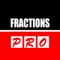# Fractions Pro

by Intemodino Group s.r.o.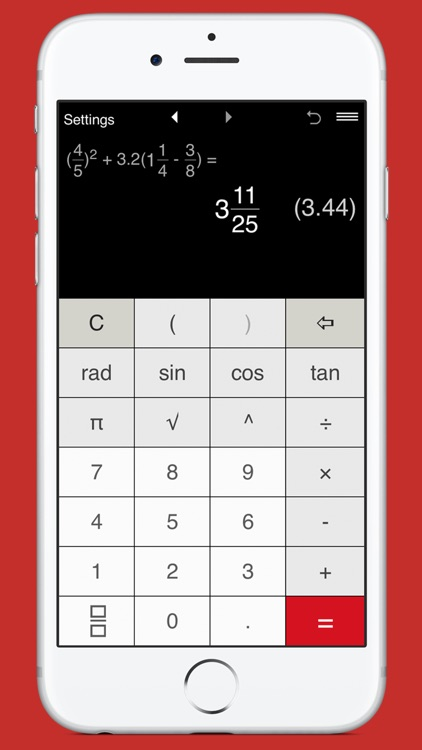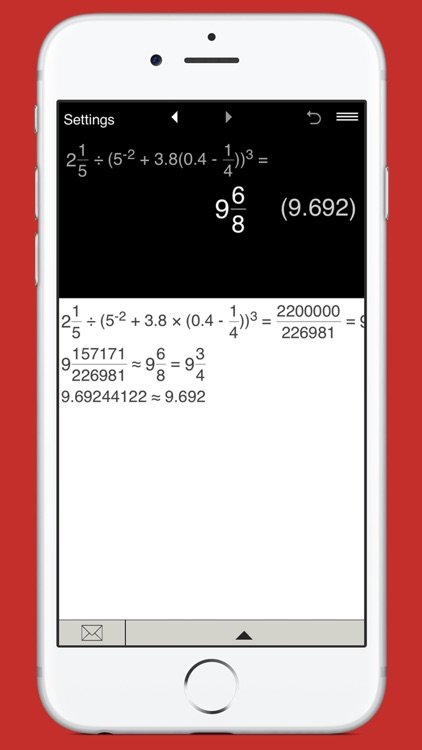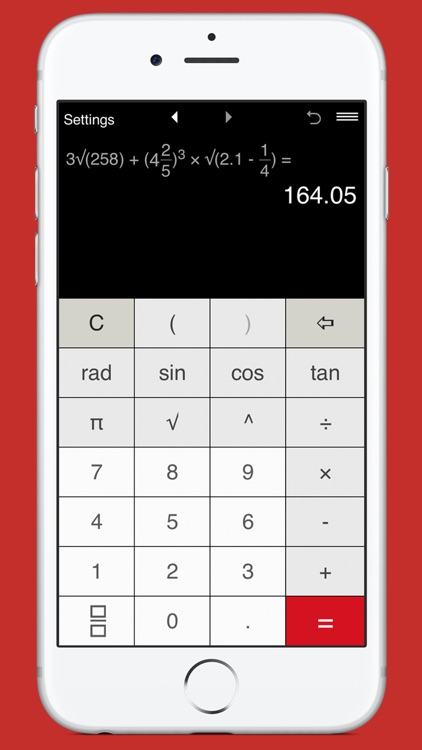Get the all-in-one multifunctional calculator with fractions, designed to perform mathematical, engineering, scientific and technical calculations of any complexity. Powerful, easy to use and well designed advanced fraction calculator lets you perform calculations with proper and improper fractions, mixed numbers, decimals and integers. Thanks to a new improved design, added intelligence and advanced functions, it is now easier than ever to quickly calculate whatever you need.### App Details

Version
2.0.2
Rating
(14)
Size
24Mb
Genre
Productivity Utilities
Last updated
September 10, 2018
Release date
November 26, 2013

### App Screenshots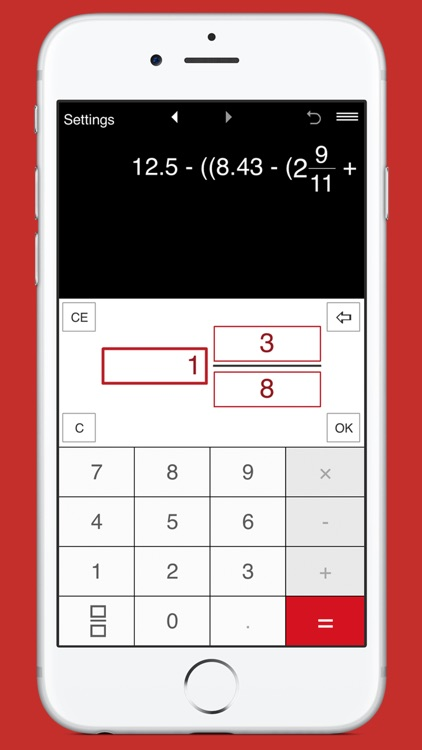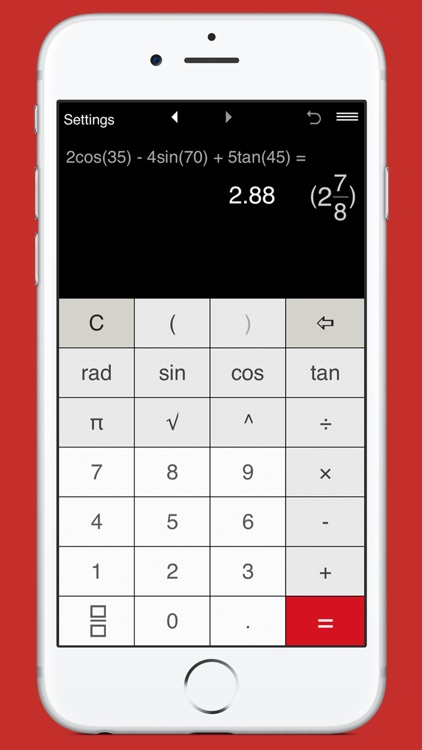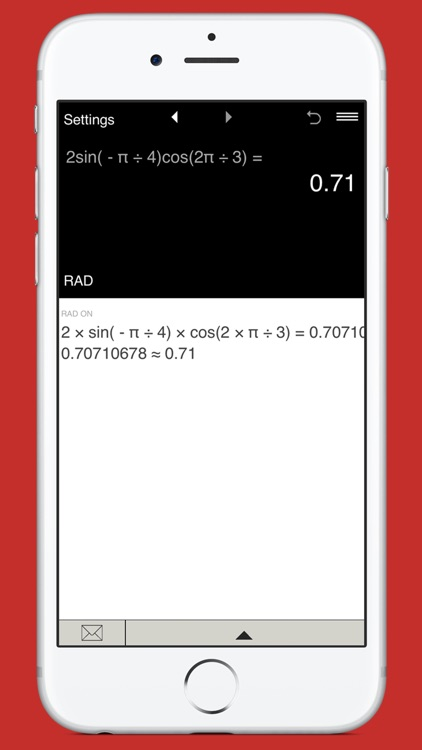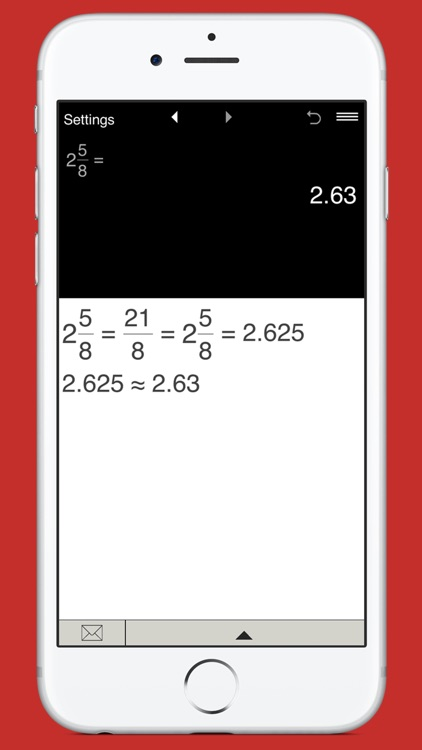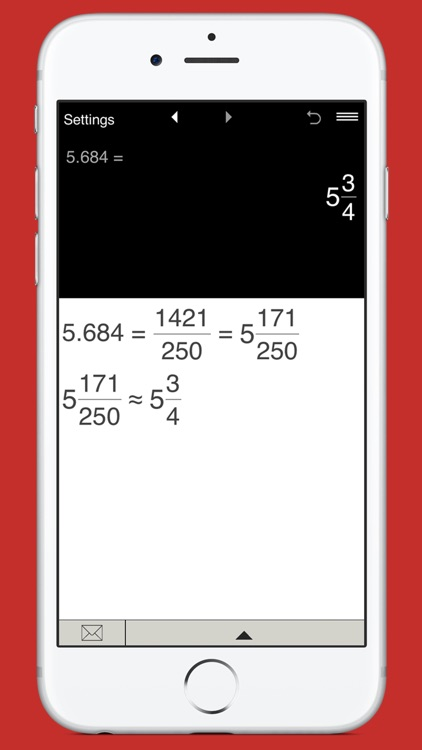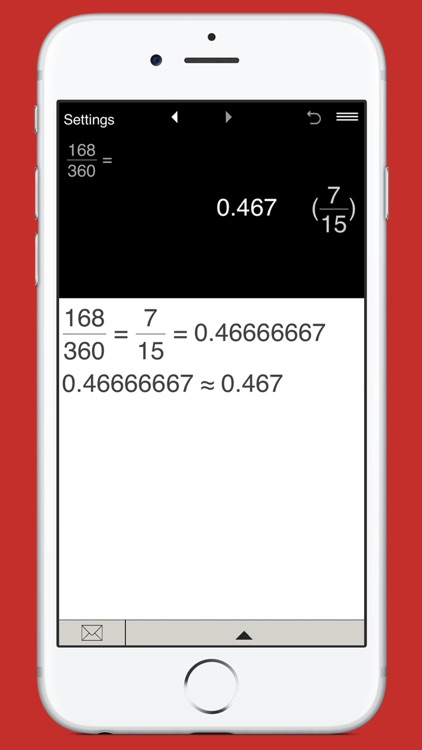### App Store Description

Get the all-in-one multifunctional calculator with fractions, designed to perform mathematical, engineering, scientific and technical calculations of any complexity. Powerful, easy to use and well designed advanced fraction calculator lets you perform calculations with proper and improper fractions, mixed numbers, decimals and integers. Thanks to a new improved design, added intelligence and advanced functions, it is now easier than ever to quickly calculate whatever you need.

Features:
- Calculations with parentheses, including nested parentheses.
- Calculations with fractions and mixed numbers.
- Intuitive fraction input.
- Exponents (integer exponents, including negative integer exponents).
- Trigonometric functions: Sin, Cos, Tan.
- Trigonometric calculations in Degrees and Radians.
- Constants: π (pi).
- Square Root (√).
- Converts fractions to decimals and decimals to fractions.
- Simplifies fractions and mixed numbers.

- Displays both Expression and Result.
- Results are displayed in both fractional and decimal formats.
- History Tape: view, edit and share calculations.
- Back and Forward buttons to check or recall recent calculations.
- Sends calculation results and history via email.
- 'Undo' for the Clear command.